Courses

# RD Sharma Solutions (Part - 1)- Ex-20.2, Mensuration - I, Class 7, Math Class 7 Notes | EduRev

## Class 7 : RD Sharma Solutions (Part - 1)- Ex-20.2, Mensuration - I, Class 7, Math Class 7 Notes | EduRev

The document RD Sharma Solutions (Part - 1)- Ex-20.2, Mensuration - I, Class 7, Math Class 7 Notes | EduRev is a part of the Class 7 Course RD Sharma Solutions for Class 7 Mathematics.
All you need of Class 7 at this link: Class 7

#### Question 1:

A rectangular grassy lawn measuring 40 m by 25 m is to be surrounded externally by a path which is 2 m wide. Calculate the cost of levelling the path at the rate of Rs 8.25 per square metre.

We have,
Length AB = 40 m and breadth BC = 25 m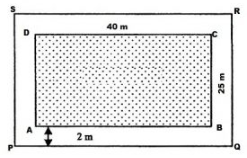∴ Area of lawn ABCD = 40 m x 25 m = 1000 m2

Length PQ = (40  + 2  + 2 ) m = 44 m
Breadth QR = ( 25 + 2 + 2 ) m = 29 m
∴ Area of PQRS = 44 m x 29 m = 1276 m2
Now,
Area of the path = Area of PQRS − Area of the lawn ABCD
= 1276 m2 − 1000 m2
= 276 m2
Rate of levelling the path = Rs. 8.25 per m2
∴ Cost of levelling the path = Rs. ( 8.25 x 276)
= Rs. 2277

#### Question 2:

One metre wide path is built inside a square park of side 30 m along its sides. The remaining part of the park is covered by grass. If the total cost of covering by grass is Rs 1176, find the rate per square metre at which the park is covered by the grass.

We have,
The side of the square garden (a) = 30 m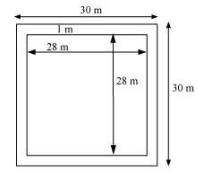∴ Area of the square garden including the path = a2 = (30)2 = 900 m2
From the figure, it can be observed that the side of the square garden, when the path is not included, is 28 m.
Area of the square garden not including the path = (28)2 = 784 m2
Total cost of covering the park with grass = Area of the park covering with green grass x Rate per square metre
1176 = 784 x Rate per square metre
∴ Rate per square metre at which the park is covered with grass = Rs. (1176 ÷ 784 )
= Rs. 1.50

#### Question 3:

Through a rectangular field of sides 90 m × 60 m, two roads are constructed which are parallel to the sides and cut each other at right angles through the centre of the field. If the which of the road is 3 m, find the total area cobered by the two roads.

We have,
Length of the rectangular field = 90 m and breadth of the rectangular field = 60 m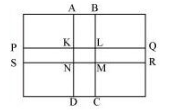∴ Area of the rectangular field = 90 m x 60 m = 5400 m2
Area of the road PQRS = 90 m x 3 m = 270 m2
Area of the road ABCD = 60 m x 3 m = 180 m2
Clearly, area of KLMN is common to the two roads.
Thus, area of KLMN = 3 m x 3 m = 9 m2
Hence,
Area of the roads = Area (PQRS) + Area (ABCD) − Area (KLMN)
= (270 + 180 )m2 − 9 m2 = 441 m

#### Question 4:

From a rectangular sheet of tin, of size 100 cm by 80 cm, are cut four squares of side 10 cm from each corner. Find the area of the remaining sheet.

We have,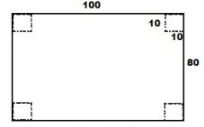Length of the rectangular sheet = 100 cm
Breadth of the rectangular sheet = 80 cm
Area of the rectangular sheet of tin = 100 cm x 80 cm = 8000 cm2
Side of the square at the corner of the sheet = 10 cm
Area of one square at the corner of the sheet = (10 cm)2 = 100 cm2
∴ Area of 4 squares at the corner of the sheet = 4 x 100 cm2 = 400 cm2
Hence,
Area of the remaining sheet of tin =Area of the rectangular sheet − Area of the 4 squares
Area of the remaining sheet of tin = (8000 − 400) cm2
= 7600 cm

#### Question 5:

A painting 8 cm long and 5 cm wide is painted on a cardboard such that there is a margin of 1.5 cm along each of its sides. Fund the total area of the margin.

We have,
Length of the cardboard = 8 cm and breadth of the cardboard = 5 cm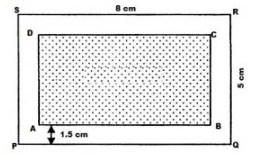∴ Area of the cardboard including the margin = 8 cm  x 5 cm = 40 cm2
From the figure, it can be observed that,
New length of the painting when the margin is not included = 8 cm − (1.5 cm + 1.5 cm) = (8 − 3) cm = 5 cm
New breadth of the painting when the margin is not included = 5 cm − (1.5 cm + 1.5 cm) = (5 − 3) cm = 2 cm
∴ Area of the painting not including the margin = 5 cm x 2 cm = 10 cm2
Hence,
Area of the margin = Area of the cardboard including the margin − Area of the painting
= (40 − 10)  cm2
= 30 cm2

#### Question 6:

Rakesh has a rectangular field of length 80 m and breadth 60 m. In it, he wants to make a garden 10 m long and 4 m broad at one of the corners and at another corner, he wants to grow flowers in two floor-beds each of size 4 m by 1.5 m. In the remaining part of the field, he wants to apply mansures. Find the cost of applying the manures at the rate of Rs 300 per are.

Length of the rectangular field  = 80 m
Breadth of the rectangular field = 60 m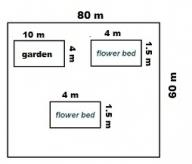∴ Area of the rectangular field = 80 m x 60
= 4800 m2
Again,
Area of the garden = 10 m x 4 m = 40 m2
Area of one flower bed = 4 m x 1.5 m = 6 m2
Thus,
Area of two flower beds = 2 x 6 m2 = 12 m2
Remaining area of the field for applying manure = Area of the rectangular field − (Area of the garden + Area of the two flower beds)
Remaining area of the field for applying manure = 4800 m2 − (40 + 12 ) m2
= (4800 − 52 )m2
= 4748 m2
Since 100 m2 = 1 are
∴ 4748 m2 = 47.48 ares

So, cost of applying manure at the rate of Rs. 300 per are will be Rs. (300 x 47.48) = Rs. 14244

Offer running on EduRev: Apply code STAYHOME200 to get INR 200 off on our premium plan EduRev Infinity!

97 docs

,

,

,

,

,

,

,

,

,

,

,

,

,

,

,

,

,

,

,

,

,

,

,

,

,

,

,

,

,

,

;